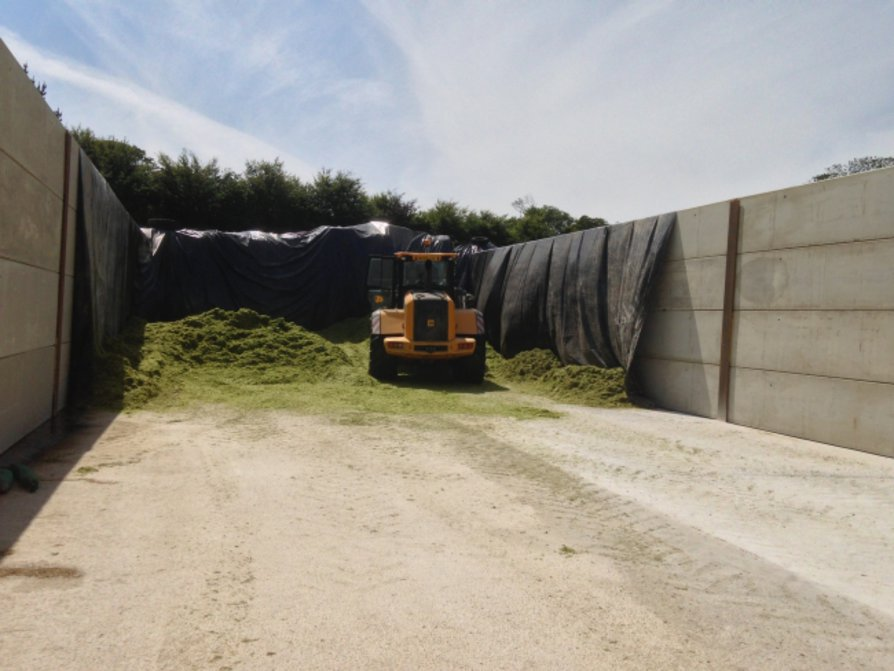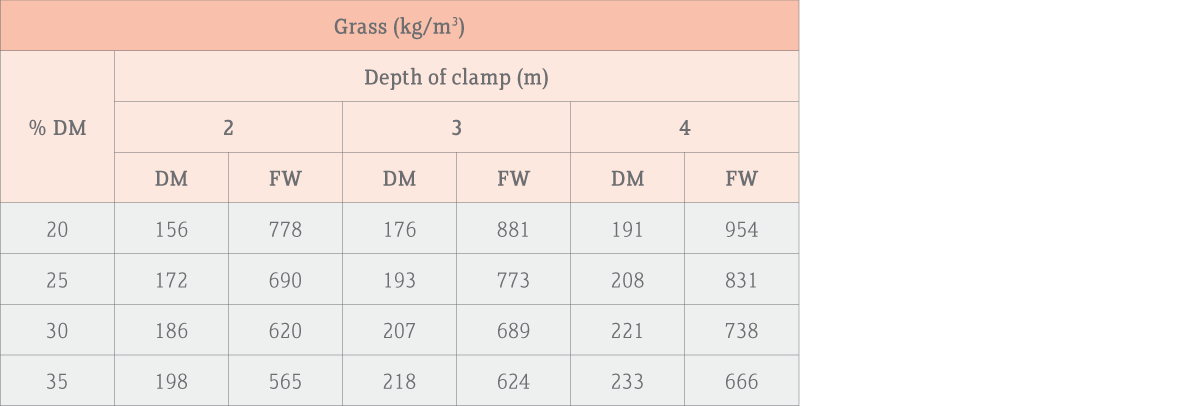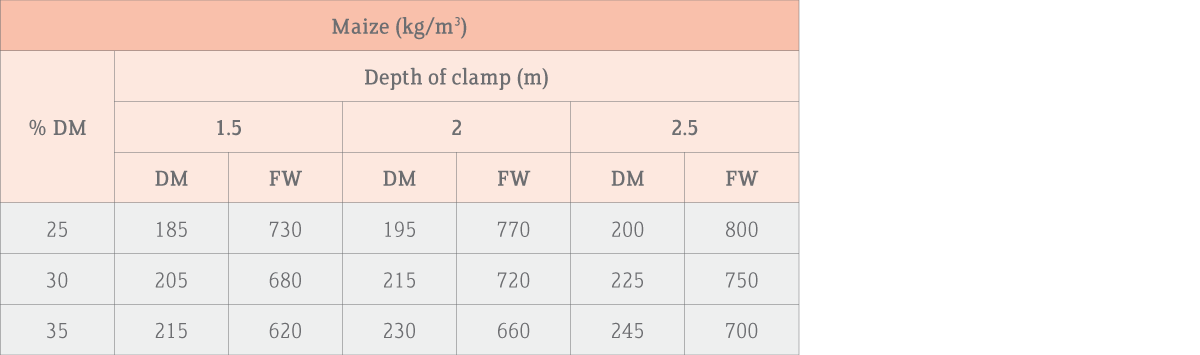# How do I calculate how much silage is in store?

### Clamps

Use our Clamp Stock Levels Tool1. Calculate the average DM of silage in the clamp as follows:

• Take several cores representing the whole clamp
• Mix the cores together very thoroughly on a clean, dry poly sheet then sub-sample.
• Weigh a suitable dish and measure exactly 100g of forage into it. Note the total weight. Repeat for 3 separate silage samples.
• Dry the sample down using a microwave oven or put it in a 60oC oven for 24 -48 hours (eg the warming oven of an Aga).
• Weigh the dish + dry sample
• Calculate the DM for each sample then average them.

%DM = (dish + dry sample) – empty dish

2. Use the silage DM and silage DM density from the tables below to tell you how much fresh silage or silage DM you would have in 1 square metre of clamp space.

3. Calculate how many metres cubes (m3) of silage you have in your clamp based on the width, length and height, eg 20m x 50m x 3m = 3,000 m3, eg
From the table, a 30% DM grass clamp with a 3m high face would contain 205 kg (0.205 tonnes) of silage DM per m3 of clamp space.

4. Calculate how much you have in the whole clamp (3,000 m3) = 900 x 0.205 = 615 tonnes of silage DM.

Depth of clamp (m)

 Grass (kg/m³) % DM Depth of clamp (m) 2 3 4 DM FW DM FW DM FW 20 156 778 176 881 191 954 25 172 690 193 773 208 831 30 186 620 207 689 221 738 35 198 565 218 624 233 666Maize (kg/m³) % DM Depth of clamp (m) 1.5 2 2.5 DM FW DM FW DM FW 25 185 730 195 770 200 800 30 205 680 215 720 225 750 35 215 620 230 660 245 700### Bales

For bales, weigh a few or estimate their weight then calculate your total fresh silage stocks. Do an oven DM on samples from several bales then calculate DM stocks as follow:

Tonnes DM = Tonnes FW x % DM ÷ 100

*DM – dry matter; FW – fresh weight

Fermented wholecrop cereal silage of 50% DM will have a freshweight density of about 600kg/m3 (200kgDM/m3).